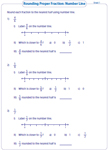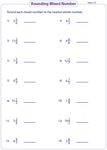# Rounding Fractions Worksheets

Rounding fraction worksheets have abundant practice skills to round the proper, improper and mixed fractions to the nearest whole number and to its nearest half. Number lines are also provided to mark and round the fraction.

Number line: Proper fraction

Indicate the given proper fraction on the number line and find if it is close to 0 or 1. Also round the fraction to the nearest whole number.Number line: Mixed number

Round each mixed number to the nearest whole number by first indicating it on the number line and thereby finding its closest value in these rounding worksheets.Number line: Nearest half - Mixed numbers

Practice each sheet by rounding the mixed number to the nearest half. Indicate the number on the number line to find the closest value.Number line: Nearest half - Proper Fractions

Learn to accurately round the proper fractions by marking it on a number line and by finding the closest half to which it is rounded.Rounding mixed number: Type 1

In section A, circle the numbers more than half and in section B circle the numbers less than half. In section C, round all the numbers to the nearest whole number.Rounding mixed number: Type 2

Rounding fractions worksheets have mixed fractions given in each sheet. Round each mixed number to the nearest whole number.Rounding improper fractions

The worksheets are based on rounding improper fraction to the nearest whole number. Before rounding, convert improper fraction into mixed number.Augment skills with the entire collection of worksheets on rounding fractions.

Related Worksheets